# Numerator/denominator indicators

### Description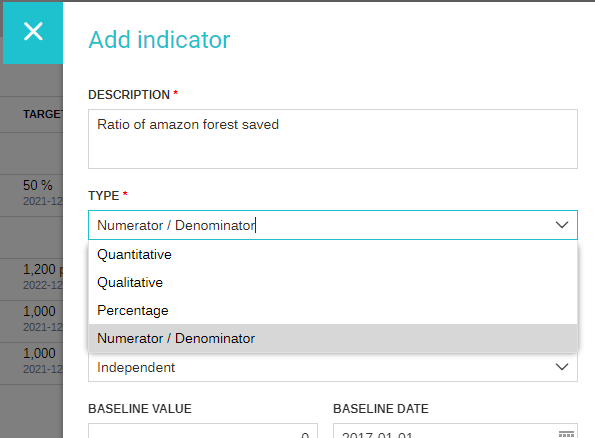When entering the actual values for this type of indicator, the user will enter the 2 values (numerator and denominator) and LogAlto will calculate the percentage value for the indicator.

##### Example

The organization is conducting a training and wants at least 80% of women participants

 Indicator % of women trained Indicator Type Numerator/Denominator Numerator Number of female participants in my training Denominator Total number of participants

In the Data Entry, when entering actual values for the indicator, the user would need to enter the Numerator (in the example: 88 female participants) and the Denominator (110 overall participants)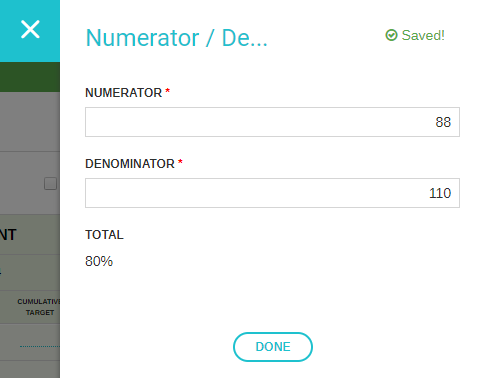The system calculated the percentage automatically from the values entered. In this case we have met the target (80% participants are women).

### Disaggregation

A numerator/dennominator indicator can be disaggregated.

The system assumes each disaggregated ratio to represent distinct but related populations.

##### Example

The organization is conducting a training and wants to track the ratio of women and men trained in a population of beneficiaries.

 Indicator % of trained beneficiaries by gender Indicator Type Numerator/Denominator Desagggregation Gender (female/male) Numerator Number of participants of specfied gender Denominator Total participants of specified gender in the beneficiary population

On a population of 100, 40 are women and 60 are men. But only 32 women (32/40) and 40 men (40/60) participated to the training.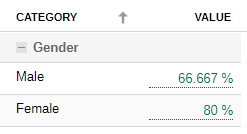Once Desegregation option is selected, 3 options would appear as follows: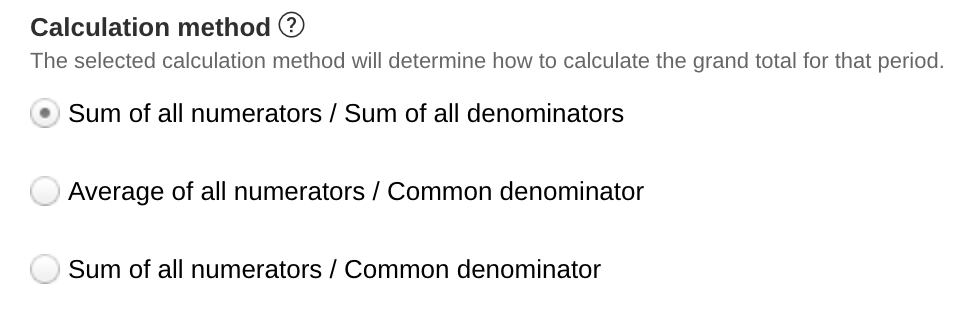The different options are explained below.

Calculations based on numerator/denominator on a disaggregated indicator

There are two calculation methods`

1
Sum of all numerators / Sum of all denominators

Proportional sum adds the numerator and denonminators of each disaggregated ratio.

With the previous example where 32/40 women and 40/60 men participated in a training, if we apply the sum then 72 out of 100 participated.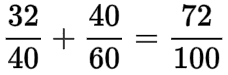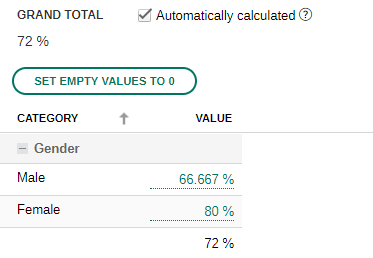2
Fractional Average of all numerators / Common
This calculation method averages the % of the ratios without considering the proportions of each disaggregation.

In effect this averages the % of each ratios, where (80%+66.66%)/2 = 73.33%.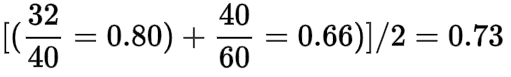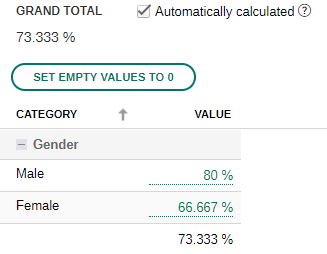3
Sum of all numerators / Common denominator
This method should be used in a progress scenario sum adds the numerator and denominators of each disaggregated ratio. his

With the previous example where 32/40 women and 40/60 men participated in a training, we had 72 out of 100 who participated. If there were 4 more women and 6 more men who participated, then to added total is 10 spots from the 100 available spots. Thus 10/100. Using this method our total is 82/100, leaving the possibility for 18 more participants, no more.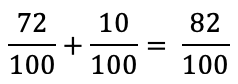`Current numerator progress + Previous numerator cumulative value * Current denominator progress / Previous denominator cumulative value`
`9+2*20/10=13`